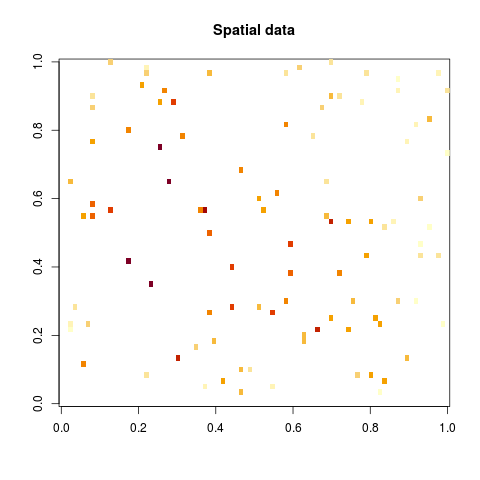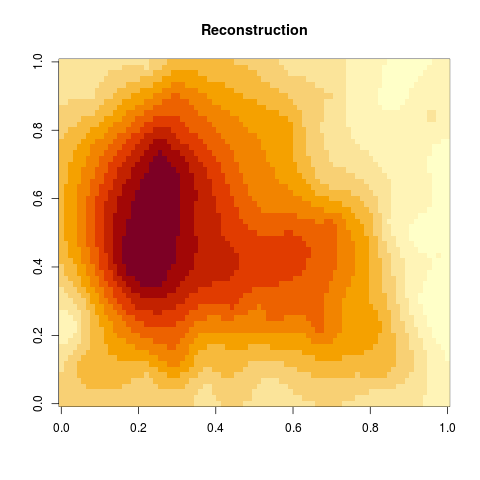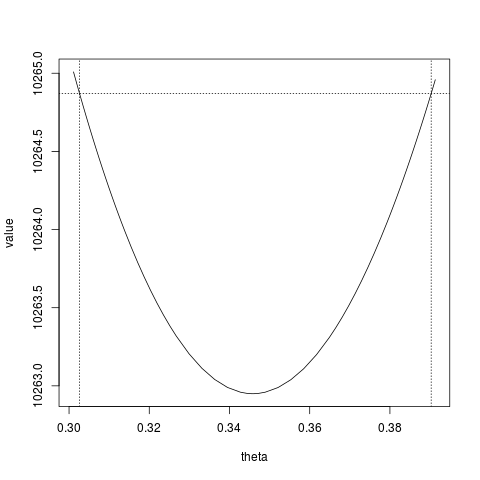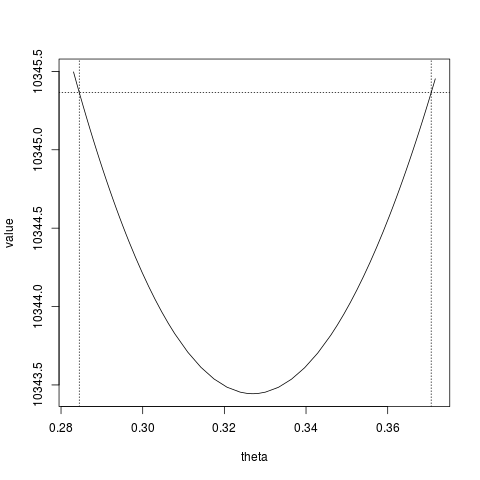# Covariance structures with glmmTMB

#### 2020-03-15

This vignette demonstrates some of the covariance structures available in the glmmTMB package. Currently the available covariance structures are:

Covariance Notation Parameter count Requirement
Heterogeneous unstructured us $$n(n+1)/2$$
Heterogeneous Toeplitz toep $$2n-1$$
Heterogeneous compound symmetry cs $$n+1$$
Heterogeneous diagonal diag $$n$$
AR(1) ar1 $$2$$
Ornstein–Uhlenbeck ou $$2$$ Coordinates
Spatial exponential exp $$2$$ Coordinates
Spatial Gaussian gau $$2$$ Coordinates
Spatial Matern mat $$3$$ Coordinates

The word ‘heterogeneous’ refers to the marginal variances of the model. Beyond correlation parameters, a heterogeneous structure uses $$n$$ additional variance parameters where $$n$$ is the dimension.

Some of the structures require temporal or spatial coordinates. We will show examples of this in a later section.

## The AR(1) covariance structure

### Demonstration on simulated data

First, let’s consider a simple time series model. Assume that our measurements $$Y(t)$$ are given at discrete times $$t \in \{1,...,n\}$$ by

$Y(t) = \mu + X(t) + \varepsilon(t)$

where

• $$\mu$$ is the mean value parameter.
• $$X(t)$$ is a stationary AR(1) process, i.e. has covariance $$cov(X(s), X(t)) = \sigma^2\exp(-\theta |t-s|)$$.
• $$\varepsilon(t)$$ is iid. $$N(0,\sigma_0^2)$$ measurement error.

A simulation experiment is set up using the parameters

Description Parameter Value
Mean $$\mu$$ 0
Process variance $$\sigma^2$$ 1
Measurement variance $$\sigma_0^2$$ 1
One-step correlation $$e^{-\theta}$$ 0.7

The following R-code draws a simulation based on these parameter values. For illustration purposes we consider a very short time series.

n <- 6                                              ## Number of time points
x <- mvrnorm(mu = rep(0,n),
Sigma = .7 ^ as.matrix(dist(1:n)) )    ## Simulate the process using the MASS package
y <- x + rnorm(n)                                   ## Add measurement noise

In order to fit the model with glmmTMB we must first specify a time variable as a factor. The factor levels correspond to unit spaced time points.

times <- factor(1:n)
levels(times)

We also need a grouping variable. In the current case there is only one time-series so the grouping is:

group <- factor(rep(1,n))

We combine the data into a single data frame (not absolutely required, but good practice):

dat0 <- data.frame(y,times,group)

Now fit the model using

glmmTMB(y ~ ar1(times + 0 | group), data=dat0)

This formula notation follows that of the lme4 package.

• The left hand side of the bar times + 0 corresponds to a design matrix $$Z$$ linking observation vector $$y$$ (rows) with a random effects vector $$u$$ (columns).
• The distribution of $$u$$ is ar1 (this is the only glmmTMB specific part of the formula).
• The right hand side of the bar splits the above specification independently among groups. Each group has its own separate $$u$$ vector but shares the same parameters for the covariance structure.

After running the model, we find the parameter estimates $$\mu$$ (intercept), $$\sigma_0^2$$ (dispersion), $$\sigma$$ (Std. Dev.) and $$e^{-\theta}$$ (First off-diagonal of “Corr”) in the output:

FIXME: Try a longer time series when the print.VarCorr is fixed.

### Increasing the sample size

A single time series of 6 time points is not sufficient to identify the parameters. We could either increase the length of the time series or increase the number of groups. We’ll try the latter:

simGroup <- function(g, n=6, rho=0.7) {
x <- mvrnorm(mu = rep(0,n),
Sigma = rho ^ as.matrix(dist(1:n)) )   ## Simulate the process
y <- x + rnorm(n)                               ## Add measurement noise
times <- factor(1:n)
group <- factor(rep(g,n))
data.frame(y, times, group)
}
simGroup(1)

Generate a dataset with 1000 groups:

dat1 <- do.call("rbind", lapply(1:1000, simGroup) )

And fitting the model on this larger dataset gives estimates close to the true values (AR standard deviation=1, residual (measurement) standard deviation=1, autocorrelation=0.7):

(fit.ar1 <- glmmTMB(y ~ ar1(times + 0 | group), data=dat1))

## The unstructured covariance

We can try to fit an unstructured covariance to the previous dataset dat. For this case an unstructured covariance has 15 correlation parameters and 6 variance parameters. Adding $$\sigma_0^2 I$$ on top would cause a strict overparameterization, as these would be redundant with the diagonal elements in the covariance matrix. Hence, when fitting the model with glmmTMB, we have to disable the $$\varepsilon$$ term (the dispersion) by setting dispformula=~0:

fit.us <- glmmTMB(y ~ us(times + 0 | group), data=dat1, dispformula=~0)
fit.us$sdr$pdHess ## Converged ?

The estimated variance and correlation parameters are:

VarCorr(fit.us)

{#1_{{#2}}} The estimated correlation is approximately constant along diagonals (apparent Toeplitz structure) and we note that the first off-diagonal is now ca. half the true value (0.7) because the dispersion is effectively included in the estimated covariance matrix (i.e. $$\rho' = \rho {2}{\sigma^2}{AR}/({2}{\sigma^2}{AR} + {2}{sigma^2}{meas})$$).

## The Toeplitz structure

The next natural step would be to reduce the number of parameters by collecting correlation parameters within the same off-diagonal. This amounts to 5 correlation parameters and 6 variance parameters.

FIXME: Explain why dispformula=~1 causes over-parameterization

fit.toep <- glmmTMB(y ~ toep(times + 0 | group), data=dat1, dispformula=~0)
fit.toep$sdr$pdHess ## Converged ?

The estimated variance and correlation parameters are:

(vc.toep <- VarCorr(fit.toep))

The diagonal elements are all approximately equal to the true total variance ($${2}{\sigma^2}{AR} + {2}{sigma^2}{meas}$$=2), and the off-diagonal elements are approximately equal to the expected value of 0.7/2=0.35.

vc1 <- vc.toep$cond[] ## first term of var-cov for RE of conditional model summary(diag(vc1)) summary(vc1[row(vc1)!=col(vc1)]) We can get a slightly better estimate of the variance by using REML estimation (however, the estimate of the correlations seems to have gotten slightly worse): fit.toep.reml <- update(fit.toep, REML=TRUE) vc1R <- VarCorr(fit.toep.reml)$cond[]
summary(diag(vc1R))
summary(vc1R[row(vc1R)!=col(vc1R)])

## Compound symmetry

The compound symmetry structure collects all off-diagonal elements of the correlation matrix to one common value.

FIXME: Explain why dispformula=~1 causes over-parameterization

fit.cs <- glmmTMB(y ~ cs(times + 0 | group), data=dat1, dispformula=~0)
fit.cs$sdr$pdHess ## Converged ?

The estimated variance and correlation parameters are:

VarCorr(fit.cs)

## Anova tables

The models ar1, toep, and us are nested so we can use:

anova(fit.ar1, fit.toep, fit.us)

ar1 has the lowest AIC (it’s the simplest model, and fits the data adequately); we can’t reject the (true in this case!) null model that an AR1 structure is adequate to describe the data.

The model cs is a sub-model of toep:

anova(fit.cs, fit.toep)

Here we can reject the null hypothesis of compound symmetry (i.e., that all the pairwise correlations are the same).

## Adding coordinate information

Coordinate information can be added to a variable using the glmmTMB function numFactor. This is necessary in order to use those covariance structures that require coordinates. For example, if we have the numeric coordinates

x <- sample(1:2, 10, replace=TRUE)
y <- sample(1:2, 10, replace=TRUE)

we can generate a factor representing $$(x,y)$$ coordinates by

(pos <- numFactor(x,y))

Numeric coordinates can be recovered from the factor levels:

parseNumLevels(levels(pos))

In order to try the remaining structures on our test data we re-interpret the time factor using numFactor:

dat1$times <- numFactor(dat1$times)
levels(dat1$times) ## Ornstein–Uhlenbeck Having the numeric times encoded in the factor levels we can now try the Ornstein–Uhlenbeck covariance structure. fit.ou <- glmmTMB(y ~ ou(times + 0 | group), data=dat1) fit.ou$sdr$pdHess ## Converged ? It should give the exact same results as ar1 in this case since the times are equidistant: VarCorr(fit.ou) However, note the differences between ou and ar1: • ou can handle irregular time points. • ou only allows positive correlation between neighboring time points. ## Spatial correlations The structures exp, gau and mat are meant to used for spatial data. They all require a Euclidean distance matrix which is calculated internally based on the coordinates. Here, we will try these models on the simulated time series data. An example with spatial data is presented in a later section. ### Matern fit.mat <- glmmTMB(y ~ mat(times + 0 | group), data=dat1, dispformula=~0) fit.mat$sdr$pdHess ## Converged ? VarCorr(fit.mat) ### Gaussian “Gaussian” refers here to a Gaussian decay in correlation with distance, i.e. $$\rho = \exp(-d x^2)$$, not to the conditional distribution (“family”). fit.gau <- glmmTMB(y ~ gau(times + 0 | group), data=dat1, dispformula=~0) fit.gau$sdr$pdHess ## Converged ? VarCorr(fit.gau) ### Exponential fit.exp <- glmmTMB(y ~ exp(times + 0 | group), data=dat1) fit.exp$sdr$pdHess ## Converged ? VarCorr(fit.exp) ### A spatial covariance example Starting out with the built in volcano dataset we reshape it to a data.frame with pixel intensity z and pixel position x and y: d <- data.frame(z = as.vector(volcano), x = as.vector(row(volcano)), y = as.vector(col(volcano))) Next, add random normal noise to the pixel intensities and extract a small subset of 100 pixels. This is our spatial dataset: set.seed(1) d$z <- d$z + rnorm(length(volcano), sd=15) d <- d[sample(nrow(d), 100), ] Display sampled noisy volcano data: volcano.data <- array(NA, dim(volcano)) volcano.data[cbind(d$x, d$y)] <- d$z
image(volcano.data, main="Spatial data", useRaster=TRUE)Based on this data, we’ll attempt to re-construct the original image.

As model, it is assumed that the original image image(volcano) is a realization of a random field with correlation decaying exponentially with distance between pixels.

Denoting by $$u(x,y)$$ this random field the model for the observations is

$z_{i} = \mu + u(x_i,y_i) + \varepsilon_i$

To fit the model, a numFactor and a dummy grouping variable must be added to the dataset:

d$pos <- numFactor(d$x, d$y) d$group <- factor(rep(1, nrow(d)))

The model is fit by

f <- glmmTMB(z ~ 1 + exp(pos + 0 | group), data=d)

Recall that a standard deviation sd=15 was used to distort the image. A confidence interval for this parameter is

confint(f, "sigma")

The glmmTMB predict method can predict unseen levels of the random effects. For instance to predict a 3-by-3 corner of the image one could construct the new data:

newdata <- data.frame( pos=numFactor(expand.grid(x=1:3,y=1:3)) )
newdata$group <- factor(rep(1, nrow(newdata))) newdata and predict using predict(f, newdata, type="response", allow.new.levels=TRUE) A specific image column can thus be predicted using the function predict_col <- function(i) { newdata <- data.frame( pos = numFactor(expand.grid(1:87,i))) newdata$group <- factor(rep(1,nrow(newdata)))
predict(f, newdata=newdata, type="response", allow.new.levels=TRUE)
}

Prediction of the entire image is carried out by (this takes a while…):

pred <- sapply(1:61, predict_col)

Finally plot the re-constructed image by

image(pred, main="Reconstruction")## Mappings

For various advanced purposes, such as computing likelihood profiles, it is useful to know the details of the parameterization of the models - the scale on which the parameters are defined (e.g. standard deviation, variance, or log-standard deviation for variance parameters) and their order.

### Unstructured

For an unstructured matrix of size n, parameters 1:n represent the log-standard deviations while the remaining n(n-1)/2 (i.e. (n+1):(n:(n*(n+1)/2))) are the elements of the scaled Cholesky factor of the correlation matrix, filled in row-wise order (see TMB documentation). In particular, if $$L$$ is the lower-triangular matrix with 1 on the diagonal and the correlation parameters in the lower triangle, then the correlation matrix is defined as $$\Sigma = D^{-1/2} L L^\top D^{-1/2}$$, where $$D = \textrm{diag}(L L^\top)$$. For a single correlation parameter $$\theta_0$$, this works out to $$\rho = \theta_0/(1+\theta_0^2)$$.

vv0 <- VarCorr(fit.us)
vv1 <- vv0$cond$group          ## extract 'naked' V-C matrix
n <- nrow(vv1)
rpars <- getME(fit.us,"theta") ## extract V-C parameters
## first n parameters are log-std devs:
all.equal(unname(diag(vv1)),exp(rpars[1:n])^2)
## now try correlation parameters:
cpars <- rpars[-(1:n)]
length(cpars)==n*(n-1)/2      ## the expected number
cc <- diag(n)
cc[upper.tri(cc)] <- cpars
L <- crossprod(cc)
D <- diag(1/sqrt(diag(L)))
round(D %*% L %*% D,3)
round(unname(attr(vv1,"correlation")),3)
all.equal(c(cov2cor(vv1)),c(fit.us$obj$env$report(fit.us$fit$parfull)$corr[]))

Profiling (experimental/exploratory):

## want $par, not$parfull: do NOT include conditional modes/'b' parameters
ppar <- fit.us$fit$par
length(ppar)
range(which(names(ppar)=="theta")) ## the last n*(n+1)/2 parameters
## only 1 fixed effect parameter
tt <- tmbprofile(fit.us$obj,2,trace=FALSE) confint(tt) plot(tt)ppar <- fit.cs$fit$par length(ppar) range(which(names(ppar)=="theta")) ## the last n*(n+1)/2 parameters ## only 1 fixed effect parameter, 1 dispersion parameter tt2 <- tmbprofile(fit.cs$obj,3,trace=FALSE)
plot(tt2)